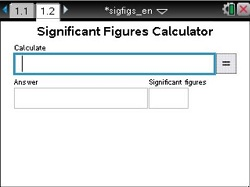••• ##### Device
•TI-Nspire™ CX/CX II
•TI-Nspire™ CX CAS/CX II CAS
• TI-Nspire™
• TI-Nspire™ CAS
• ##### Software

TI-Nspire™
TI-Nspire™ CAS

4.2

# Chemistry: Tool - Significant Figures Calculator

by Texas Instruments - Skills of science Lesson#### Objectives

This document allows you to perform calculations using addition (+), subtraction (-), multiplication (*), and division (/).  It lets you display the significant figures of entered values and perform mathematical operations using either decimal or scientific notation and display the result with the correct number of significant digits.

#### About the Lesson

This tool can be used alone, or you can copy/paste it into another TNS document.  When you perform calculations that involve measurements, the answer can be no more precise than the least precise measurement.  A conventional calculator does not consider precision; it shows results with the maximum number of digits it can display.  To maintain the precision of the measurements, these calculated results should be rounded to the correct number of significant figures.